• 掌握Encoder-Decoder框架
• 掌握残差网络
• 掌握BatchNormalization(批归一化)和LayerNormalization(层归一化)
• 掌握Position Embedding(位置编码)

Notes: 本文代码参考哈弗大学的The Annotated Transformer

## Encoder-Decoder框架

Encoder-Decoder是为seq2seq（序列到序列）量身打造的一个深度学习框架，在机器翻译、机器问答等领域有着广泛的应用。这是一个抽象的框架，由两个组件：Encoder（编码器）和Decoder(解码器)组成。对于给定的输入source $(x_1, x_2, x_3, ...,x_n)$, 首先编码器将其编码成一个中间表示向量$z=(z_1, z_2, ..., z_n)$。接着，解码器根据z和解码器自身前面的输出，来生成下一个单词（如Figure 1所示）。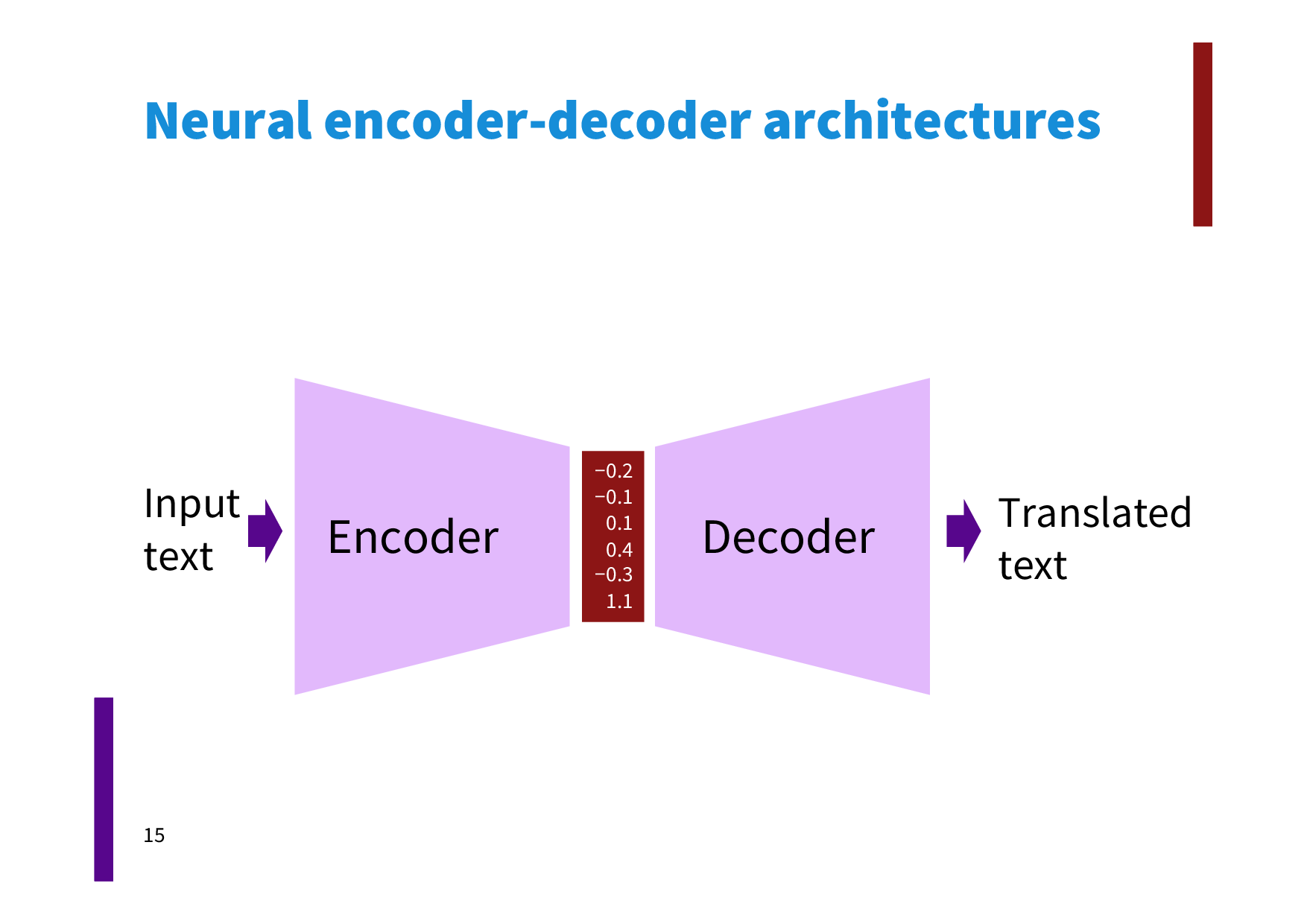Figure 1

## 整体架构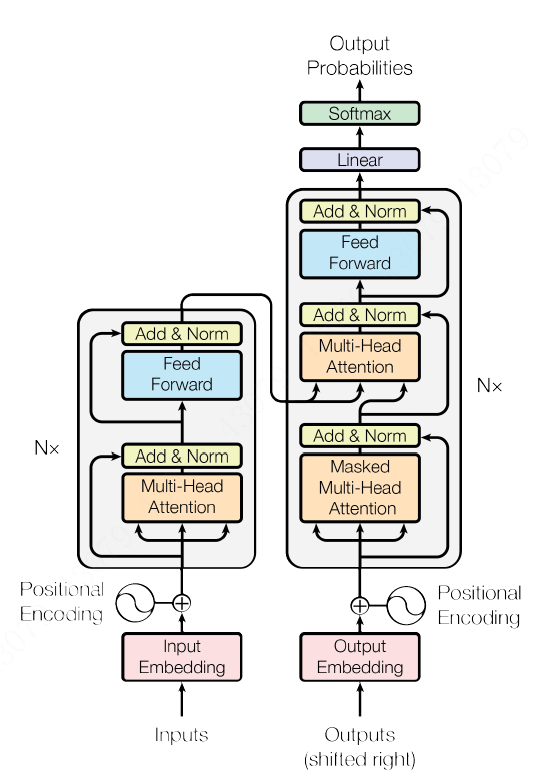Figure 2

## Encoder

### EncoderLayer和残差网络

EncoderLayer如Figure 3所示。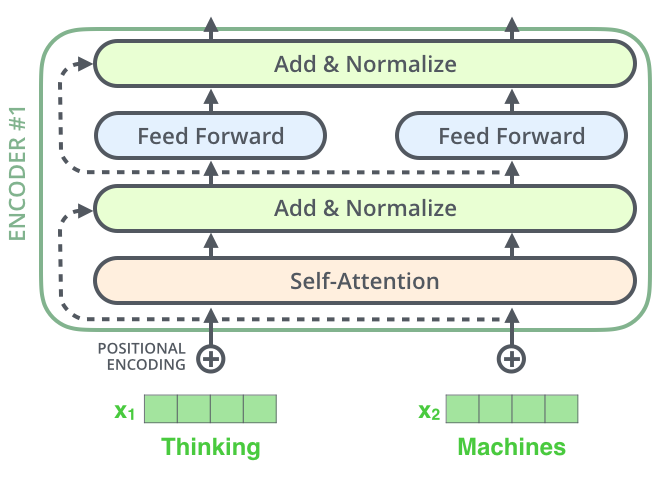Figure 3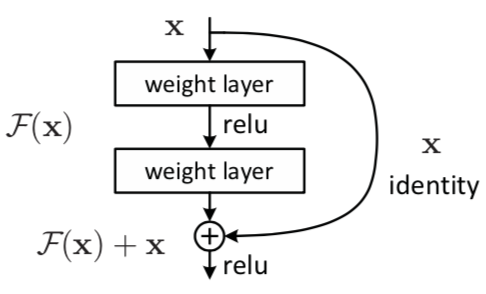Figure 4

• 输入x
• x做一个层归一化： x1 = norm(x)
• 进入多头self-attention: x2 = self_attn(x1)
• 残差加成：x3 = x + x2
• 再做个层归一化：x4 = norm(x3)
• 经过前馈网络: x5 = feed_forward(x4)
• 残差加成: x6 = x3 + x5
• 输出x6

### 多头注意力机制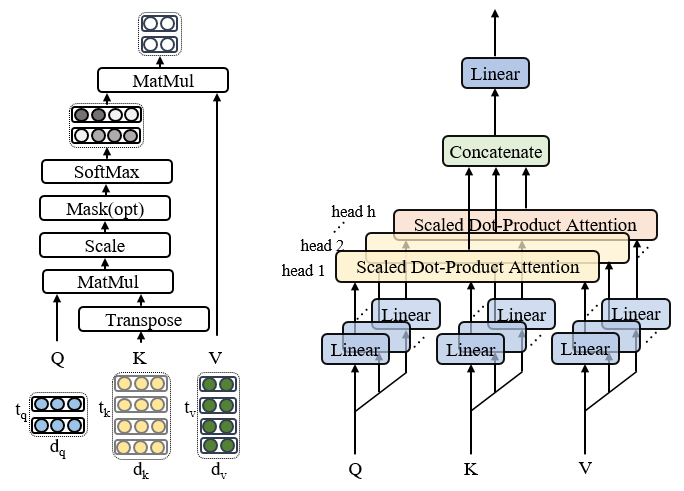• 一是Encoder的输出.
• 二是target的前面的单词.

### 层归一化

#### BatchNormalization

$z = g(Wu+b)$

$x=Wu+b$

$z = sigmoid(x) = g(x) = \frac{1}{1+exp(-x)}$

$\frac{\partial z}{\partial W} = \frac{\partial z}{\partial g}\cdot\frac{\partial g}{\partial x}\cdot\frac{\partial x}{\partial u}$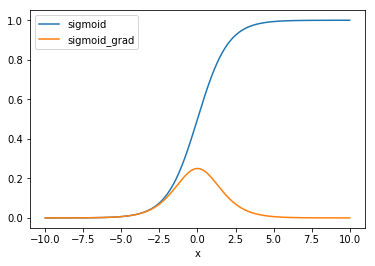Figure 5
BatchNormalization就是解决这两个问题的。首先，它将隐藏层的输入强行变换为同一分布（解决了第一个问题），这个分布就是正态分布（解决了第二个问题）。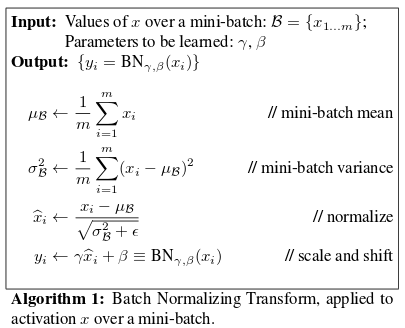Figure 6

#### LayerNormalization

BatchNormalization简直是个救世主啊，它令调参工作变得从未如此容易，让调参侠们不费吹灰之力，谈笑间到达收敛的彼岸。但毛主席曾经说过，万物都是辩证的，它同样存在两个问题：

• 对batch_size非常敏感。BatchNormalization的一个重要出发点是保持每层输入的数据同分布。回想下开始那个独立同分布的假设。假如取的batch_size很小，那显然有些Mini-Batch的数据分布就很可能与整个数据集的分布不一致了，又出现了那个问题，数据分布不一致，这就等于说没起到同分布的作用了，或者说同分布得不充分。实验也证明，batch_size取得大一点， 数据shuffle的好一点，BatchNormalization的效果就越好。

• 不能很方便地用于RNN。这其实是第一个问题的引申。我们再来看一下Figure 6中的均值和方差的计算公式。对所有样本求均值。对于图片这类等长的输入来说，这很容易操作，在每个维度加加除除就可以了，因为维度总是一致的。而对于不等长的文本来说，RNN中的每个time step共享了同一组权重。在应用BatchNormalization时，这就要求对每个time step的batch_size个输入计算一个均值和方差。那么问题就来了，假如有一个句子S非常长，那就意味着对S而言，总会有个time_step的batch_size为1，均值方差没意义，这就导致了BatchNormalization在RNN上无用武之地了。

LayerNormalization的主要变化在于：

• 不再对Mini-Batch中的N的样本在各个维度做归一化，而是针对同一层的所有神经元做归一化。归一化公式为：

$\mu^l = \frac{1}{H}\Sigma_1^Ha_i^l$

$\sigma^l = \sqrt{\frac{1}{H}\Sigma_1^H(a_i^l-\mu^l)}$

其中，H指的是一层神经网络的神经元个数。我们再回想下BatchNormalization，其实它是在每个神经元上对batch_size个数据做归一化，每个神经元的均值和方差均不相同。而LayerNormalization则是对所有神经元做一个归一化，这就跟batch_size无关了。哪怕batch_size为1，这里的均值和方差只和神经元的个数有关系（如果读到这里仍然感到不是特别清楚，再读两遍，还困惑也没关系，待会看Figure 7）。

• 测试的时候可以直接利用LN，所以训练时不用保存均值和方差，这节省了内存空间。

Figure 7示意了两种方式的区别。假设有N个样本，每个样本的特征维度为4，图中每个小圆代表一个特征，特征1，特征2等等，特征4。BatchNormalization是在N个同一特征（如特征1）上求均值和方差，这里要对每个特征求1次，共4次。对照一下上面说的，万一有个样本有5个特征，是不是就没法玩了。LayerNormalization呢，别的样本都和我没啥关系，有多少个特征我把这些特征求个均值方差就好了。这也就是为什么一个叫”批归一化“，另一个叫”层归一化“了。理解了这一点，也就理解了为什么Transformer中使用LN而不是BN。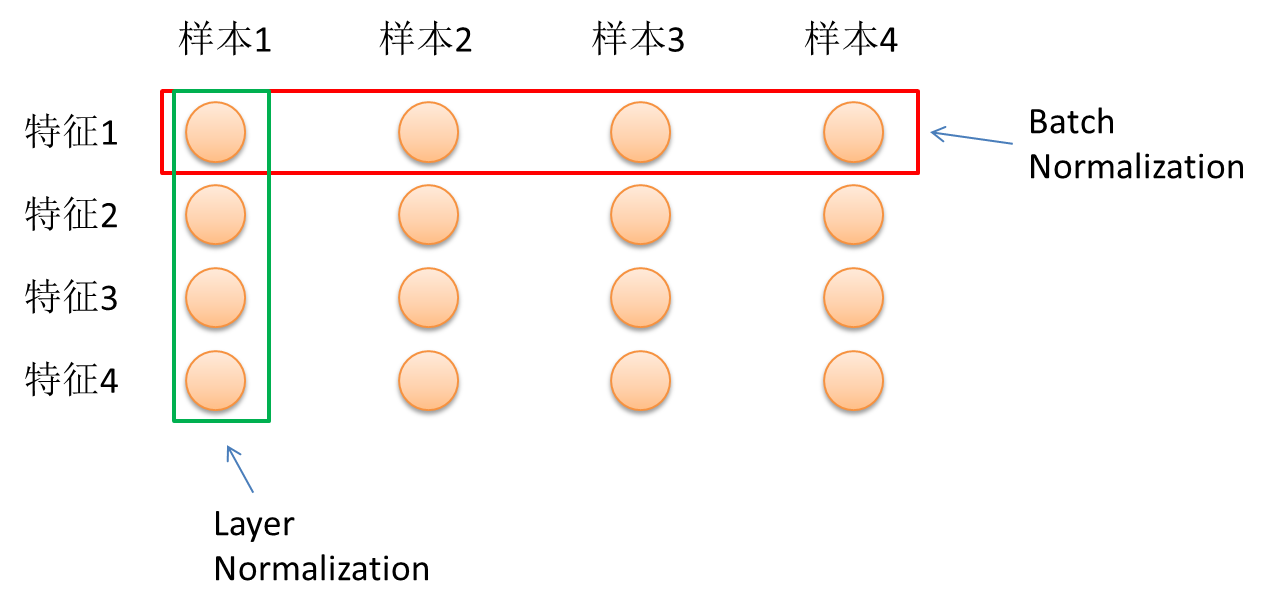Figure 7

### 前馈网络

$\mathrm{FFN}(x)=\max(0, xW_1 + b_1) W_2 + b_2$

## 词向量

### 位置编码

$PE_{(pos,2i)} = sin(pos / 10000^{2i/d_{\text{model}}})$

$PE_{(pos,2i+1)} = cos(pos / 10000^{2i/d_{\text{model}}})$

$PE_{(pos,0)} = sin(pos)$

$sin(i)=sin(j)$

$i, j $为非负整数且$i$不等于$j$

$i=(-1)^k\cdot j+k\cdot{\pi}$

$i, j $为非负整数且$i$不等于$j$且$k$为整数

$\pi=\frac{[i-(-1)^k\cdot j]}{k}$

$PE(1, 0)=cos(1)$

$PE(k,0) = sin(1+k) = sin(1)cos(k)+cos(1)sin(k) = A+B\cdot PE(1,0)$

## Deocder